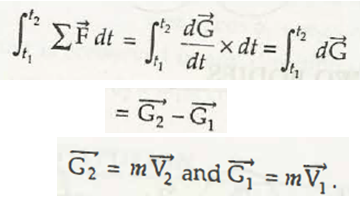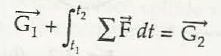Linear Impulse can be defined as the equivalent change in linear momentum in the same direction. The S.I. unit of Linear Impulse is Newton-second (N-s).

Let us consider force acting on the body be

Linear momentum can be given by,dt

Suppose the force acts on the body between separate interval of time t1 and t2. Now, linear momentum can be given by,

It can be modified as,

= dt

= 21

Where,

2= m 2

1 = m 1Components of Linear Impulse

Considering the components of linear impulse, it consists of x, y and z components. These are written as-

xdt = m x2 – m x1

ydt = m y2 – m y1

zdt = m z2 – m z1

Links of Next Mechanical Engineering Topics:-### Customer Reviews

My Homework Help
Rated 5.0 out of 5 based on 510 customer reviews at